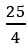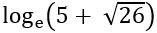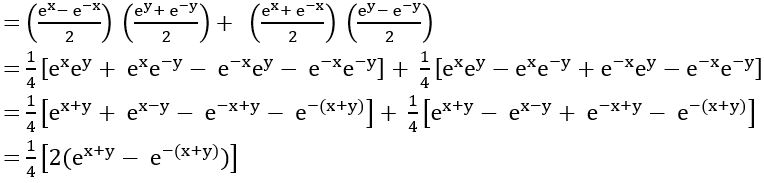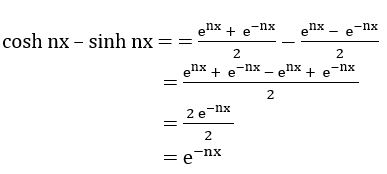Welcome To Basics In Maths

# Hyperbolic Functions V.S.A.Q.’S# These solutions designed by the ‘Basics in Maths‘ team. These notes to do help the intermediate First-year Maths students.

## Inter Maths – 1A two marks questions and solutions are very useful in IPE examinations.

Hyperbolic Functions

#### Question 1

Prove that for any x∈ R, sinh (3x) = 3 sinh x + 4 sinh3 x

Sol:

sinh (3x) = sinh (2x + x)

= sinh 2x cosh x + cosh 2x sinh x

= (2 sinh x cosh x) cosh x + (1 + 2 sinh2 x) sinh x

= 2sinh x cosh2 x + sinh x + 2 sinh3 x

= 2 sinh x (1 + sinh2 x) + sinh x + 2 sinh3 x

= 2 sinh x + 2 sinh3 x+ sinh x + 2 sinh3 x

= 3 sinh x + 4 sinh3 x

#### Question 2

If cosh x =, find the values of (i) cosh 2x and (ii) sinh 2x

Sol:

Cosh 2x = 2 cosh2 x – 1

Sinh2 2x = cosh2 2x – 1

Sol:

#### Question 4

If sinh x = 5, then show that x =Sol:

Given, sinh x = 5

⟹ x = sinh-15

Sol:

Given tanh-1

##### Question 6

For x, y ∈ R prove that sinh (x + y) = sinh (x) cosh (y) + cosh (x) sinh (y)

Sol:

R.H.S = sinh (x) cosh (y) + cosh (x) sinh (y)= sinh (x + y)

##### Question 7

For any x∈ R, prove that cosh4 x – sinh4 x = cosh 2x

Sol:

cosh4 x – sinh4 x = (cosh2 x)2 – (sinh2 x)2

= (cosh2 x + sinh2 x) (cosh2 x – sinh2 x)

= 1. cosh 2x

= cosh 2x

##### Question 8

Sol:

= cosh x + sinh x

##### Question 9

If sin hx = ¾ find cosh 2x and sinh 2x.

Sol:

Given sin hx = ¾

We know that cosh2 x = 1 + sinh2 x

= 1 + (3/4)2

= 1 + 9/16

= 25/16

cos hx = 5/4

cosh 2x = 2cosh2 x – 1

= 2(25/16) – 1

= 25/8 – 1

= 17/8

Sinh 2x = 2 sinh x cosh x

= 2 (3/4) (5/4)

= 15/8

#### Question 10

Prove that (cosh x – sinh x) n = cosh nx – sinh nx

Sol:∴ (cosh x – sinh x) n = cosh nx – sinh nx

#### Visit My YouTube Channel:  Click on below logo